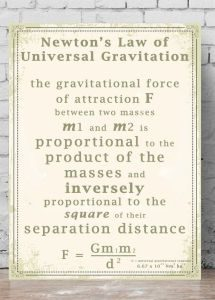# Dynamics – Newton’s Laws

0
3608

They studies of movement dates since the ancient Greece. For instance, Aristotle had an opinion that the bodies moves and that movement was created as a consequence of the natural order of things, such as that the natural place for the air was up and for the earth was down.

Later on the body movement was studied by a large number of naturalists, but the most significant contribution was given by Galileo Galileo. He noticed that bodies keep their speed unchanged if there was no interaction woth them.So far we studied mechanical movements without taking into account the cause of their movements. I this section we will study the movements and causes that led to movement and the interaction between the bodies.

We have already mentioned what is the cause of interaction in nature, and now we will introduce new quantities such as mass, force, and impulse of the body.
Anything that happens in nature is the consequence of the interaction between the bodies. The type of a certain body movement depends on the strength, direction and duration of the force that caused it, as well as the mechanical movement.The basic laws of dynamics were formulated by Newton in 1687 in his work “The Mathematical Basis of Natural Philosophy” and these laws are called Newton’s laws or laws of movement. Newton’s laws are objective laws of nature, established on the basis of observations and experiments of Newton himself and his predecessors.

The relationships between the kinetamic quantities and the dynamics quantities are all given by the basic laws of mechanics, which was precisely formulated by Newton, which is why it is called the Newton’s Mechanics.

The following are Newton’s laws:

1. Newton’s First Law: The Law of Inertion: http://aziza-physics.com/en/newtons-first-law-the-law-of-inertion/

2. Newton’s Second Law: http://aziza-physics.com/en/newtons-second-law-the-law-of-motion/

3. Newton’s Third Law: The Law of Action and Reaction:  http://aziza-physics.com/en/third-newton-law-the-law-of-action-and-reaction/

4. Newton’s Law of Gravitation: http://aziza-physics.com/en/newtons-law-of-gravity/SHARE
Previous articleThe Basis of Thermodynamics